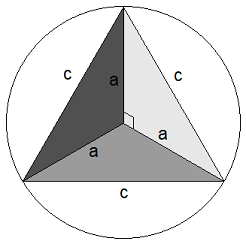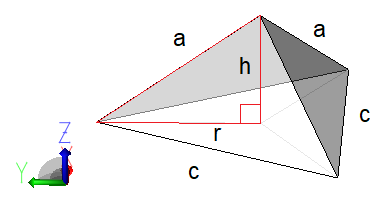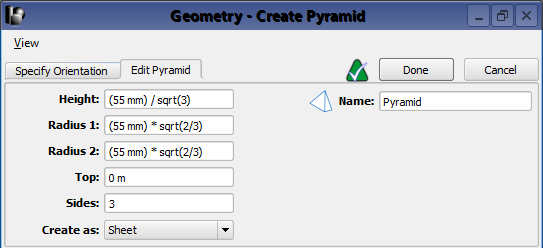# Corner Reflector Equations | WaveFarer

Geometric relationship to a pyramid defined.A corner reflector is modeled as a pyramid in WaveFarer. The following geometric formulas express the relationship between a corner reflector's inner edge lengths and a pyramid's radius and height.

• $a$: length of a corner reflector's inner edge.
• $c = \sqrt{2}a$: length of an edge along the corner reflector's open face.
• $r = \sqrt{\frac{2}{3}}a$: radius of the circle that circumscribes the triangle with three edges of length $c$.
• $h = a/\sqrt{3}$: distance between the center of the corner reflector's open face and its inner vertex.

## Background

These equations assume that the corner reflector consists of three faces of equal size. Each face is a triangle with one 90 degree angle and two 135 degree angles. Given an inner length of $a$, the Pythagorean theorem is used to determine the length of an edge along the corner reflector's open face, $c = \sqrt{2}a$. WaveFarer defines a pyramid in terms of its radius and height, so these values' relationship to $a$ and $c$ must be determined.The pyramid's radius, $r$, defines a circle that circumscribes the equilateral triangle with edges of length $c$. The radius is determined by splitting the equilaterial triangle into three isosceles triangles.

\begin{eqnarray} \cos(30^\circ) &=& \frac{c/2}{r} \\ \frac{\sqrt{3}}{2} &=& \frac{c/2}{r} \\ r &=& \frac{c}{\sqrt{3}} \\ r &=& \sqrt{\frac{2}{3}}a \end{eqnarray}The pyramid's height, $h$, is the distance between the center of the equilaterial triangle with edges of length $c$ and the corner reflector's inner vertex. The Pythagorean theorem is used to determine $h$ once $r$ has been determined.

\begin{eqnarray} h^2 + r^2 &=& a^2 \\ h^2 + \frac{2a^2}{3} &=& a^2 \\ h^2 &=& \frac{a^2}{3} \\ h &=& a/\sqrt{3} \end{eqnarray}

## Pyramid ControlsCreate a pyramid by right-clicking on Parts in the Project Tree, and selecting Create New ❯ Pyramid to open the pyramid editor.

Three of the editing fields should always use the following settings:

• Top: 0
• Sides: 3
• Create as: Sheet

The remaining fields can be specified as equations, such as in the following examples that assume the corner reflector has an inner edge length of 55 mm:

• Height: (55 mm) / sqrt(3)
• Radius 1: (55 mm) * sqrt(2/3)
• Radius 2: (55 mm) * sqrt(2/3)

A parameter can also be entered into the fields, such as in the following equations that use parameter innerLength set to 55 mm:

• Height: innerLength / sqrt(3)
• Radius 1: innerLength * sqrt(2/3)
• Radius 2: innerLength * sqrt(2/3)

Click Done to create a four-sided pyramid. Then right-click on Pyramid in the Project Tree and select Modify ❯ Remove Faces to create a three-sided corner reflector.